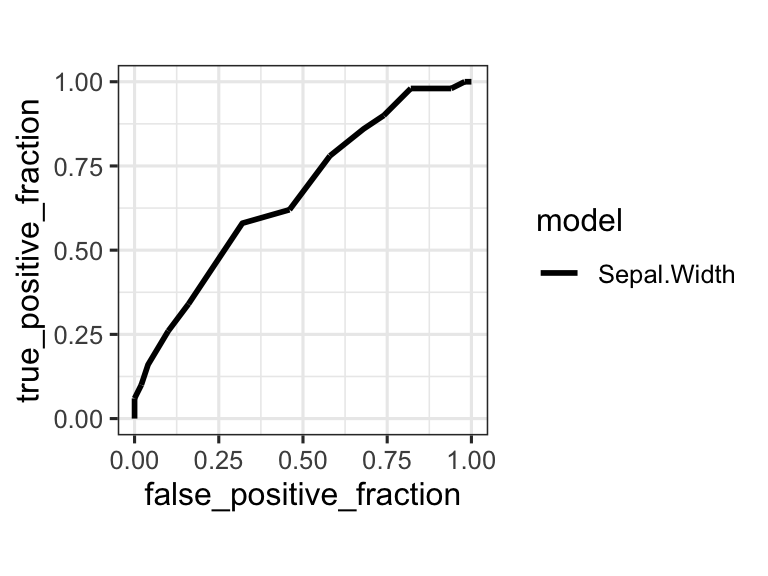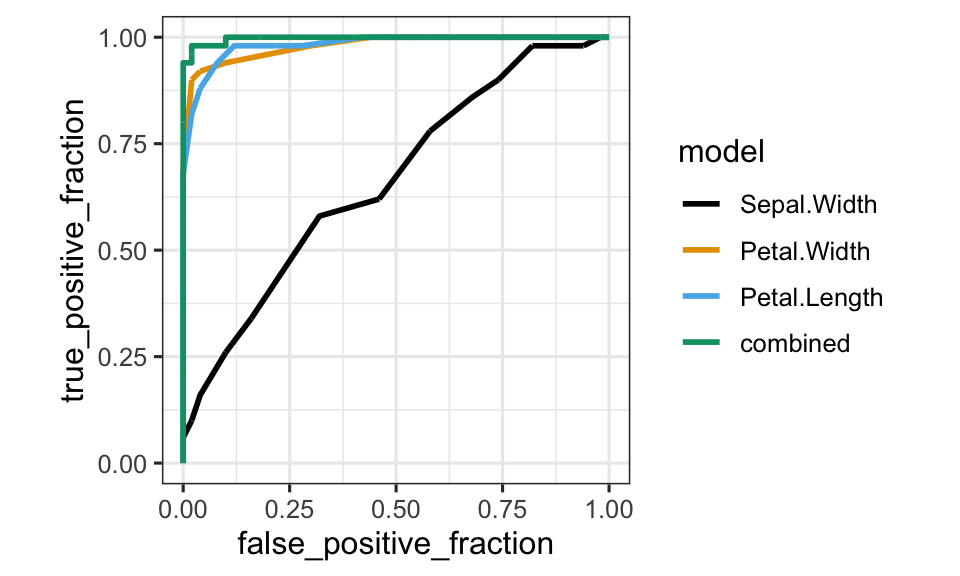## In-class worksheet 12

Feb 27, 2020

In this worksheet, we will use the libraries tidyverse, ggthemes, and plotROC:

library(tidyverse)
theme_set(theme_bw(base_size = 12)) # set default ggplot2 theme
library(ggthemes) # for scale_color_colorblind()
library(plotROC) # for geom_roc() and calc_auc()

# 1. True positive and true negative rates

We continue working with the two species virginica and versicolor from the iris data set. As was done in the previous class, the following code makes a reduced data set, fits a logistic regression model (this time using only Sepal.Length as predictor), and then combines the predicted probabilities and the known species identity into one data frame called pred_data:

# make a reduced iris data set that only contains virginica and versicolor species
iris_small <-
iris %>%
filter(Species %in% c("virginica", "versicolor")) %>%
mutate(Species = factor(Species)) # this makes R forget about the 3rd species

# fit logistic regression model
glm_out <- glm(
Species ~ Sepal.Length,
data = iris_small,
family = binomial
)

# combine fitted values and Species identity
pred_data <- data.frame(
probability = glm_out$fitted.values, Species = iris_small$Species
)

Pick a probability cutoff at which you would decide that a specimen belongs to virginica rather than versicolor. Calculate how many virginicas you call correctly and how many incorrectly given that cutoff. Hint: use the functions filter() and tally().

cutoff <- 0.5 # cutoff of 0.5
virg_true <-
pred_data %>%
filter(probability >= cutoff & Species=="virginica") %>%
tally()
virg_true
##    n
## 1 37
virg_false <-
pred_data %>%
filter(probability < cutoff & Species=="virginica") %>%
tally()
virg_false
##    n
## 1 13

Now do the same calculation for versicolor.

vers_true <-
pred_data %>%
filter(probability < cutoff & Species=="versicolor") %>%
tally()
vers_true 
##    n
## 1 36
vers_false <-
pred_data %>%
filter(probability >= cutoff & Species=="versicolor") %>%
tally()
vers_false
##    n
## 1 14

If we define a call of virginica as a positive and a call of versicolor as a negative, what are the true positive rate (sensitivity, true positives divided by all possible positives, i.e., by the total count of virginicas) and the true negative rate (specificity, true negatives divided by all possible negatives, i.e., by the total count of versicolors) in your analysis?

# get total counts
virg_total <-
pred_data %>%
filter(Species=="virginica") %>%
tally()

vers_total <-
pred_data %>%
filter(Species=="versicolor") %>%
tally()

# true positive rate
tp <- virg_true$n / virg_total$n
tp
##  0.74
# true negative rate
tn <- vers_true$n / vers_total$n
tn
##  0.72

The true-positive rate (sensitivity) is 0.74 and the true-negative rate (specificity) is 0.72.

Recalculate the true-positive rate and the true-negative rate for a different probability cutoff.

cutoff <- 0.1 # cutoff of 0.1
virg_true <-
pred_data %>%
filter(probability >= cutoff & Species=="virginica") %>%
tally()

vers_true <-
pred_data %>%
filter(probability < cutoff & Species=="versicolor") %>%
tally()

# true positive rate
tp <- virg_true$n/(virg_total$n)
tp
##  0.98
# true negative rate
tn <- vers_true$n/(vers_total$n)
tn
##  0.08

At a cutoff of 0.1, we get a true-positive rate of 0.98 and a true-negative rate of 0.08.

# 2. ROC curves

Next we will calculate and plot ROC curves. We do this with the function geom_roc() from the package plotROC. Here is an example:

# fit the model
glm_out <- glm(
Species ~ Sepal.Width,
data = iris_small,
family = binomial
)

# make data frame of the linear predictor and the known truth
df1 <- data.frame(
predictor = predict(glm_out, iris_small), # linear predictor
known_truth = iris_small$Species, # the known truth, i.e., true species assignment model = "Sepal.Width" # an arbitrary name to distinguish different curves ) # now plot using geom_roc() # the aesthetic names are not the most intuitive # d (disease) holds the known truth # m (marker) holds the predictor values ggplot(df1, aes(d = known_truth, m = predictor, color = model)) + geom_roc(n.cuts = 0) + coord_fixed() + scale_color_colorblind() ## Warning in verify_d(data$d): D not labeled 0/1, assuming versicolor = 0 and
## virginica = 1!Now make a few additional models and then make a plot showing the various ROC curves in one graph. (Hint: combine the data frames from the different models into one using the function rbind().)

glm_out <- glm(
Species ~ Petal.Width,
data = iris_small,
family = binomial
)
df2 <- data.frame(
predictor = predict(glm_out, iris_small),
known_truth = iris_small$Species, model = "Petal.Width" ) glm_out <- glm( Species ~ Petal.Length, data = iris_small, family = binomial ) df3 <- data.frame( predictor = predict(glm_out, iris_small), known_truth = iris_small$Species,
model = "Petal.Length"
)

glm_out <- glm(
Species ~ Petal.Width + Petal.Length + Sepal.Width,
data = iris_small,
family = binomial
)
## Warning: glm.fit: fitted probabilities numerically 0 or 1 occurred
df4 <- data.frame(
predictor = predict(glm_out, iris_small),
known_truth = iris_small$Species, model = "combined" ) df_combined <- rbind(df1, df2, df3, df4) ggplot(df_combined, aes(d = known_truth, m = predictor, color = model)) + geom_roc(n.cuts = 0) + coord_fixed() + scale_color_colorblind() ## Warning in verify_d(data$d): D not labeled 0/1, assuming versicolor = 0 and
## virginica = 1!# 3. If this was easy

Calculate the area under the ROC curve (AUC) for your first model, using the function calc_auc() from the plotROC package. This function needs to be called on a plot containing a geom_roc() statement.

# make plot and save as p
p <- ggplot(df1, aes(d = known_truth, m = predictor, color = model)) +
geom_roc(n.cuts = 0)

# calculate AUC
calc_auc(p)
## Warning in verify_d(data$d): D not labeled 0/1, assuming versicolor = 0 and ## virginica = 1! ## PANEL group AUC ## 1 1 1 0.6636 Now calculate the AUC values for all the ROC curves you generated earlier, using the function calc_auc() exactly once. p <- ggplot(df_combined, aes(d = known_truth, m = predictor, color = model)) + geom_roc(n.cuts = 0) calc_auc(p) ## Warning in verify_d(data$d): D not labeled 0/1, assuming versicolor = 0 and
## virginica = 1!
##   PANEL group    AUC
## 1     1     1 0.6636
## 2     1     2 0.9804
## 3     1     3 0.9822
## 4     1     4 0.9972

Unfortunately, because of how ggplot works, we have now lost the model names and instead just have group numbers. We can recover the connection between model name and group number by calling unique(df_combined$model) to obtain the model names and order(unique(df_combined$model)) to obtain the corresponding group numbers. Use this information to generate a modified table that contains model name and AUC and is sorted in descending order of AUC.

model <- unique(df_combined$model) model_info <- data.frame( model, group = order(model) ) left_join(model_info, calc_auc(p)) %>% select(-group, -PANEL) %>% arrange(desc(AUC)) ## Warning in verify_d(data$d): D not labeled 0/1, assuming versicolor = 0 and
## virginica = 1!
## Joining, by = "group"
##          model    AUC
## 1     combined 0.9972
## 2 Petal.Length 0.9822
## 3  Petal.Width 0.9804
## 4  Sepal.Width 0.6636